1800-1023-196

+91-120-4616500

# Molecular Collisions

## Molecular Collisions

(1) The closest distance between the centres of two molecules taking part in a collision is called molecular or collision diameter (s). The molecular diameter of all the gases is nearly same lying in the order of 10-8m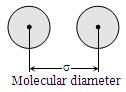(2) The number of collisions taking place in unit time per unit volume, called collision frequency (z).

(i) The number of collision made by a single molecule with other molecules per unit time are given by, ZA = √2πσ2uav.n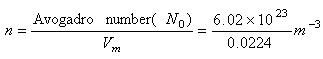Where n is the number of molecules per unit molar volume,

(ii) The total number of bimolecular collision per unit time are given by,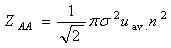(iii) If the collisions involve two unlike molecules, the number of bimolecular collision are given by,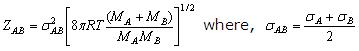MA, MB are molecular weights (M = mN0)

(iv) (a) At particular temperature; Z∝P2

(b) At particular pressure; Z∝ T-3/2

(c) At particular volume; Z∝ T1/2

(3) During molecular collisions a molecule covers a small distance before it gets deflected. The average distance travelled by the gas molecules between two successive collision is called mean free path (λ).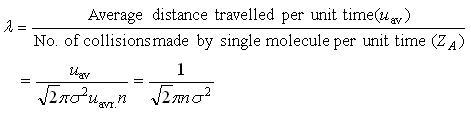(4) Based on kinetic theory of gases mean free path, λ∝(T/P). Thus,

(i) Larger the size of the molecules, smaller the mean free path, i.e., λ∝ (1/(radius)2)

(ii) Greater the number of molecules per unit volume, smaller the mean free path.

(iii) Larger the temperature, larger the mean free path.

(iv) Larger the pressure, smaller the mean free path.

(5) Relation between collision frequency (Z) and mean free path (l) is given by, Z = Urms/ λ

## NEET & AIIMS Exam Sample Papers

 AIIMS SAMPLE PAPERS View More NEET SAMPLE PAPERS View More